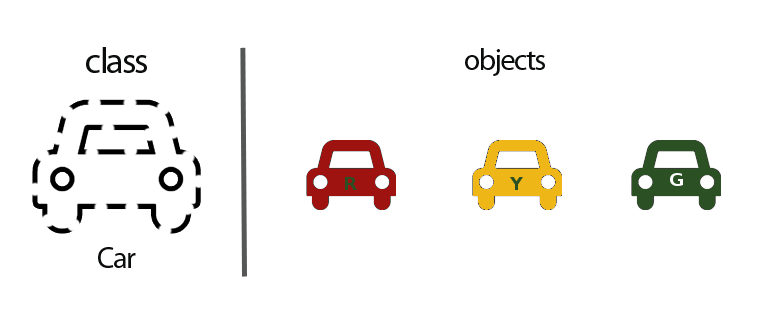### Argument - Parameter

A parameter (or formal argument) is a variable in a function or method definition. When the function is called, the argument is the data we pass in the function call.

  int square(int number)  // number is the parameter
{ return number * number; }
---
auto result = square(3); // 3 is the argument


### Callback

A callback is a function that is passed as an argument to a function, which is expected to call back (execute) at a convenient time. This may for instance be to display information (as the number of lines) of a file once read.

  // Callback definition
function displayCount(count)
{ console.log("File contains " + count + " lines."); }

readFile("text.txt", displayCount); // Callback passed as argument

Callbacks are used everywhere in association with events in web development. In the case of an asynchronous call, call back can be executed when a response has been received.

### Class

A class (prototype) is a template that defines object properties and may also describe object behavior.class Car // Class definition
{
public:
Car(string color) { this.color = color; } // Constructor
string GetColor() { return this.color; }  // Method
private:
string color;  // Attribute
}

const auto greenCar = new Car('green'); // Create instance
cout << greenCar.GetColor();            // Display car color - 'green'


We can think of class as a sketch (prototype) of a car. It contains all the details about the number of tires, the doors, the color... Based on these descriptions we built a green car. As many cars can be made from the same prototype, we can create as many objects as we want from a class.

### Constructor

The constructor is a method, inside a class, that is used to instantiate (create an instance) an object. It is automatically called when an object is built.

### Curried function

A curried function is a function that takes multiple arguments and returns successively another function that will take the next argument provided as a parameter. The last function returns the result of applying the function to all of its arguments.

  const sum = x => y => x + y;
sum (2);    // returns a function y => 2 + y
sum (2)(1); // returns 3

In other words, it is a function that takes multiple arguments one at a time.

### Function

A function is a named section of a program that performs a specific task.

  multiply(3, 3); // multiply is the function called
Some programming languages make a distinction between a function, which returns a value, and a procedure, which performs some operation but does not return a value.

### Instance

An instance is an occurrence of a class. Above (cf. Class), greenCar is an instance of the class Car. Another instance of this class could be created by writing:

  const auto otherGreenCar = new Car('green');

### Method

A method is a function that belongs to a class or an object. It is bound to it, defined only within that scope of usage.

### Nested Function

A nested function is a function contained inside of another function. It can be used to limit the scope of the inner function.

function getSurfBoard(weather, size, height) {
const boardList = getBoardForWeather(weather);

// Nested function
function filterBoardByBody(size, height) {...}
}


### Recursive Function

The term recursive describes a function or method that calls itself.

Understanding how recursion works is a fundamental programming skill.
function fibonacci(number) {
if (number === 0) return 0;
if (number === 1) return 1;
return fibonacci(number - 1) + fibonacci(number - 2);
}

As a reminder the Fibonacci numbers are defined as follow:
F(0) = 0
F(1) = 1
F(n) = F(n−1) + F(n−2)